Select Page

# 12 Science Maths CBSE Solutions for MCQ Relations and Functions in English

12 Science Maths CBSE Solutions for MCQ Relations and Functions in English to enable students to get Solutions in a narrative video format for the specific question.

Expert Teacher provides 12 Science Maths CBSE Solutions for MCQ Relations and Functions through Video Solutions in English language. This video solution will be useful for students to understand how to write an answer in exam in order to score more marks. This teacher uses a narrative style for a question from Relations and Functions not only to explain the proper method of answering question, but deriving right answer too.

Please find the question below and view the Solution in a narrative video format.

Question:

Solution Video in English:

You can select video Solutions from other languages also. Please check Solutions in ( Hindi )

## Similar Questions from CBSE, 12th Science, Maths, Relations and Functions

Question 1 : Letbe defined as f(x) = 3x. Choose the correct answer. (View Answer Video)

Question 2 : Let A ={1, 2, 3}. Then number of equivalence relations containing (1, 2) is: (View Answer Video)

Question 3 : Letdefined as f(x) = x be an identity function. Then, (View Answer Video)

Question 4 :  Let * be any binary operation on the set R defined by a * b = a + b – ab, then the binary operation * is, (View Answer Video)

Question 5 :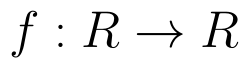be  defined as f(x) =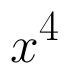(View Answer Video)

### Linear Programming

Question 1 : The objective function is maximum or minimum, which lies on the boundary of the feasible region. (View Answer Video)

### Differential Equations

Question 1 : Find the differential equation of the family of lines passing through the origin.  (View Answer Video)

Question 2 : Find the particular solution of the differential equation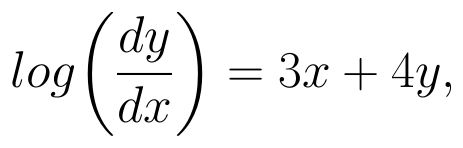given that y = 0, when x = 0. (View Answer Video)

Question 3 : Write the differential equation formed from the equation y = mx + c, where m and c are arbitrary constants.

Question 4 : Write the degree of the differential equation :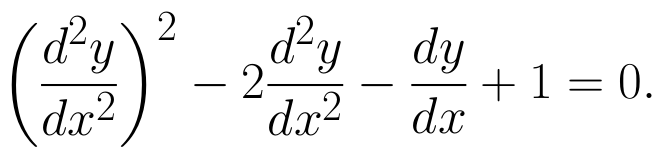(View Answer Video)

Question 5 : Solve the differential equation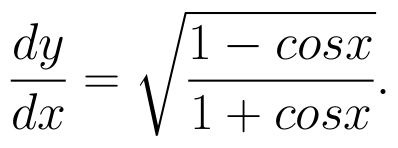(View Answer Video)

### Continuity and Differentiability

Question 1 : Findfor the function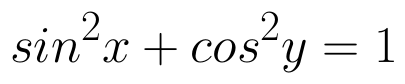. (View Answer Video)

Question 2 : Differentiate the function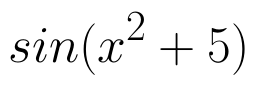with respect to x. (View Answer Video)

Question 3 : If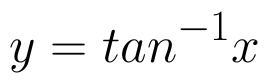, find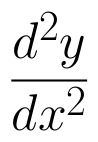in terms of y alone. (View Answer Video)

Question 4 : Findfor the function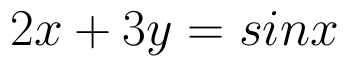. (View Answer Video)

Question 5 :  Find the second order derivative of the function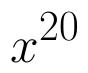. (View Answer Video)